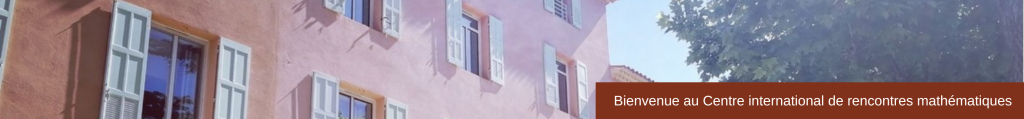WORKSHOP​Fusibles NumbersNombres fusibles 14 – 18 June 2021
Description
The set of fusible numbers is the least set of rationals such that 0 is fusible and for fusible x,y if |x-y|<1, then the number (x+y+1)/2 is fusible. There is a less formal description of this set as the set of all intervals of time measurable by a certain procedure using so-called fuses. Recently Erickson, Nivasch, and Xu have proved that the true statement « for any rational q there is the nearest to the right fusible number » is independent from first-order Peano arithmetic. For any monotone function f(x_1,..,x_n) we consider the set G_f that is the least set such that 0∈G_f and for any x_1,…,x_n∈G_f if f(x_1,…,x_n)>max(x_1,…,x_n), then f(x_1,…,x_n)∈G_f. The sets G_f could be regarded as generalizations of the set of fusible numbers that itself corresponds to the case of f(x,y)=(x+y+1)/2.  We investigate the  the order types of the sets G_f
Participants

Konstantin Bogdanov (Aix-Marseille Université)
Alexander Bufetov (Aix-Marseille Université)
Pierre Lazag (Aix-Marseille Université)
Juan Marshall (Aix-Marseille Université)
Gabriel Nivasch (Ariel University)
Fedor Pakhomov  (
Steklov Mathematical Institute of Russian Academy of Sciences Moscow)
Université Paris-Est Marne-la-Vallée)

(Marseille, France local time) https://www.thetimezoneconverter.com

Monday 14 June
14.00  Gabriel Nivasch (Ariel University)
Fusible numbers and Peano Arithmetic   (abstract)

Wednesday 16 June
15.15  Alexander Bufetov (CNRS & Institut de Mathématiques de Marseille & Steklov, IITP RAS)
Determinantal point processes: quasi-symmetries, minimality and interpolation   (abstract)